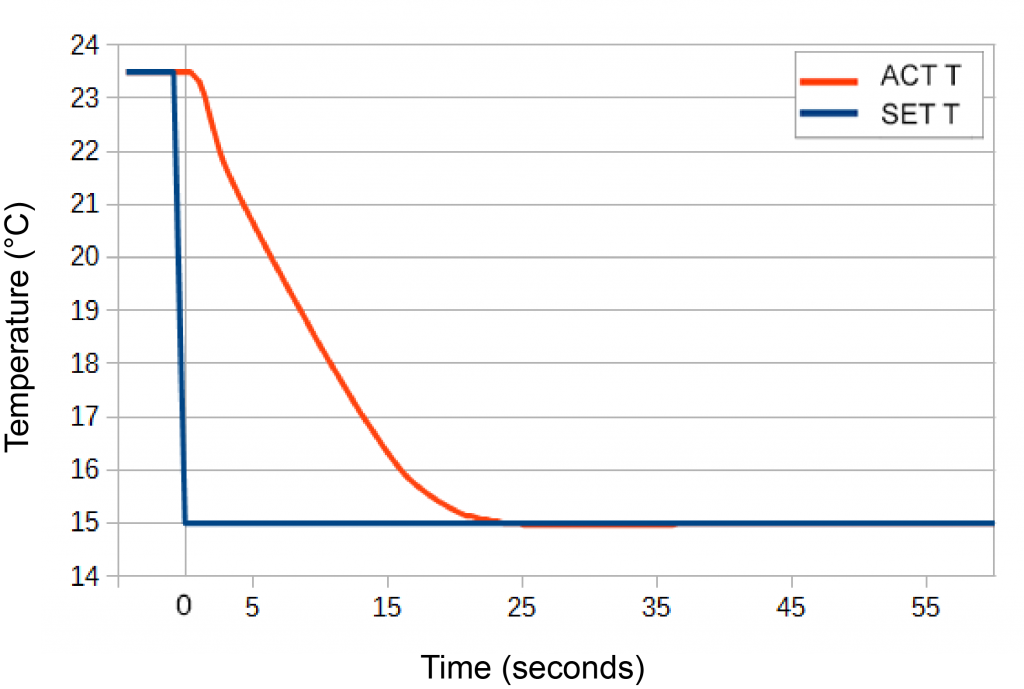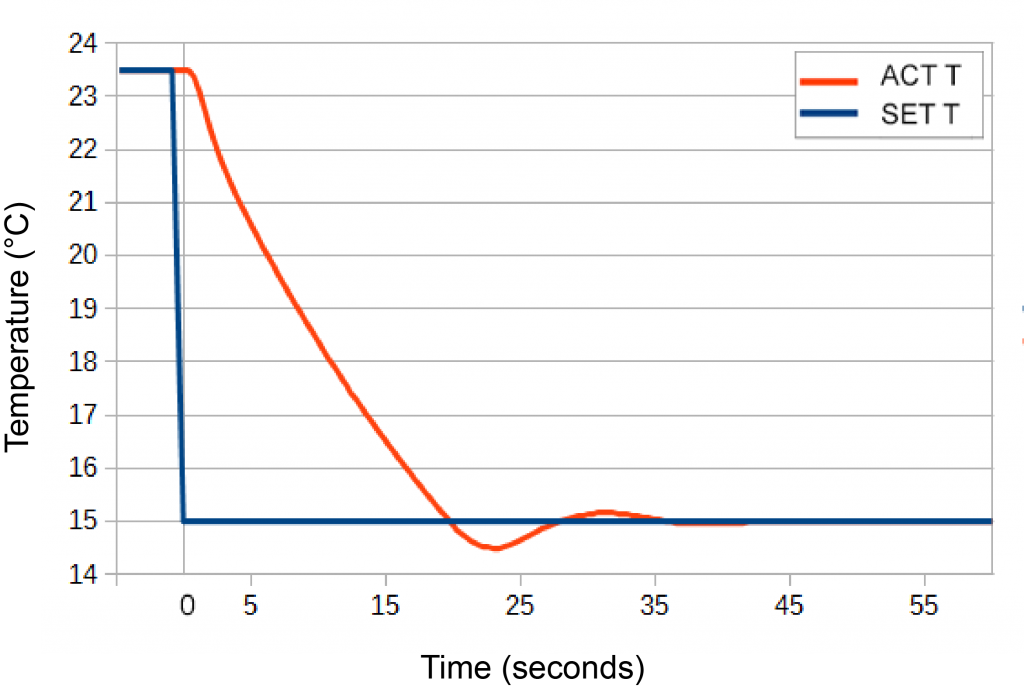TC10 LAB
TC15 LAB

## Question

How can I reduce time to temperature with the TC LAB Series instruments?

A strategy Wavelength often suggests to reduce time to temperature is to push the control system to a point where it is over-damped, where any overshoot is within the tolerance parameter. We manually iterated the P and D terms by factors ranging from 1.89 to 2.1 to find this point. We put the TC10 LAB into Manual Tuning mode and forced PID coefficients of 46.820, 0.929, and 4.452 (2.1 times the P and D coefficients in Disturbance Rejection mode at 15°C). The following response was observed.Figure 1. Manual Tuning, 22.8 seconds to stable temperature First crossing of setpoint: 24.0 seconds P=46.820; I=0.929; D=4.452Figure 2. Tuned, 39.9 seconds to stable temperature First crossing of setpoint: 19.9 seconds P=14.081; I=1.408; D=1.594 Setpoint Response

The time to first crossing increased from 19.9 seconds (Figure 2) to 24.0 seconds (Figure 1). Because there was no overshoot, the temperature was in tolerance at 22.8 seconds, instead of 39.9 seconds.

In some instances, overdamping the system sacrifices steady state performance and stability is not as good. For the TC10 LAB and this load, stability in Disturbance Rejection mode or overdamped mode was comparable.